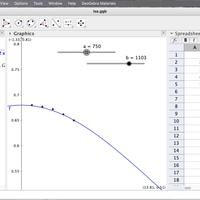# Best fit curves in GeoGebraSome of my students and I are having difficulty plotting curves in Loggerpro. There seems to be some bugs. Curve plotting is necessary in the IA when an equation can not be linearised, however it is important that the curve plotted is based on some theoretical model and not just some random curve that fits. Personally I prefer not to linearize, plotting the curve gives a much better feel for the relationship.

The example I use on the video is Isa's IA. She is investigating the relationship between the distance from the centre and the angular velocity of a mass sliding along a rotating bar. The conservation of angular momentum says that the angular momentum of the beam + mass is constant

Iω + mr2ω = k

So

ω = k/(I + mr2)

Here is a video explaining how to use GeoGebra to plot a best fit curve based on this equation. This method can of course also be used for straight lines.